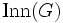# Every automorphism is center-fixing and inner automorphism group is maximal in automorphism group implies every automorphism is normal-extensible

## Statement

Suppose$G$ is a group with center$Z(G)$, inner automorphism group$\operatorname{Inn}(G)$, and automorphism group$\operatorname{Aut}(G)$. Suppose the following two statements are true:

• Every automorphism of$G$ is a center-fixing automorphism: it fixes every element of the center$Z(G)$.
•$\operatorname{Inn}(G)$ is a maximal subgroup of$\operatorname{Aut}(G)$.

Then, every automorphism of$G$ is a normal-extensible automorphism: whenever$G$ is a normal subgroup of some group$K$ and$\sigma$ is an automorphism of$G$,$\sigma$ extends to an automorphism$\sigma'$ of$K$.

## Facts used

1. Center-fixing implies central factor-extensible: If$\sigma$ is a center-fixing automorphism of a central factor$G$ of a group$K$,$\sigma$ extends to an automorphism of$K$.
2. Equivalence of definitions of central factor

## Proof

Given: A group$G$ such that every automorphism of$G$ fixes every element of$Z(G)$, and$\operatorname{Inn}(G)$ is a maximal subgroup of$\operatorname{Aut}(G)$.

To prove: For any automorphism$\sigma$ of$G$, and any group$K$ containing$G$ as a normal subgroup,$\sigma$ extends to an automorphism of$K$.

Proof: Let$\alpha:K \to \operatorname{Aut}(G)$ be the homomorphism given by the action of$K$ on$G$ by conjugation. This map exists because$G$ is normal in$K$.

1. The image of$\alpha$ is either$\operatorname{Inn}(G)$ or$\operatorname{Aut}(G)$: The image of$\alpha$ is a subgroup of$\operatorname{Aut}(G)$. It contains$\operatorname{Inn}(G)$, because$\operatorname{Inn}(G)$ equals$\alpha(G)$. Since$\operatorname{Inn}(G)$ is a maximal subgroup of$\operatorname{Aut}(G)$, the image is either$\operatorname{Inn}(G)$ or$\operatorname{Aut}(G)$.
2. If the image is$\operatorname{Aut}(G)$, then every automorphism of$G$ extends to an inner automorphism of$K$, and we are done. (We say in this case that$G$ is a normal fully normalized subgroup of$K$).
3. If the image is$\operatorname{Inn}(G)$, then$G$ is a central factor of$K$, i.e.,$K = G C_K(G)$ (see fact (2)). Thus, by fact (1), any automorphism$\sigma$ that fixes the center of$G$ extends to an automorphism of$K$. Since we assumed that every automorphism fixes the center of$G$, we obtain that every automorphism of$G$ extends to an automorphism of$K$.